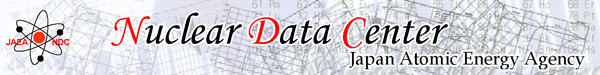Plotting Tool for ENDF  ~ Usage ~

1. Initial status
For the first time the page is opened, initial values below have been set in columns for input, and a graph of U-235 is shown.
[Libraries] = JENDL-4.0 (300K),  [Nuclides] = U-235,  [MT Numbers] = 1, 102
[X-axis Type] = log,  [X-axis Range] = 1.0e-5 to 2.0e+7
[Y-axis Type] = log,  [Y-axis Range] = “Auto” is set.
2. Setting parameters
The parameters on the left side of the page can be changed to set graph status as the table below.
 Libraries Select library and temperature. Nuclides Input nuclides in “[Element name] (-) [Mass] (Meta stable state [m])” format. Cross sections of natural elements are given by mass number = 0 . Natural abundances are taken from Ref. .  P. De Bihre and P.D.P. Taylor, Int. J. Mass Spectrom. Ion Processes 123, 149-166 (1993). A hyphen between the element name and the mass isn't necessary. Both capital and small letter are allowed for alphabetical input. More than one nuclide should be separated by comma (,) characters. MT Numbers Input MT numbers correspond to nuclear reactions in the ENDF-6 format. The table of MT numbers vs nuclear reactions can be seen. X-axis Type Select “Linear” or “Log” for X-axis scale. X-axis Range Input range (the upper and lower boundary) of X-axis in eV, or check “Auto” to make the range set automatically. Y-axis Type Select “Linear” or “Log” for Y-axis scale. Y-axis Range Input range (the upper and lower boundary) of Y-axis in barns, or check “Auto” to make the range set automatically.
3. Redrawing the graph
The graph is redrawn with the set condition after clicking the “Draw Graph” button.